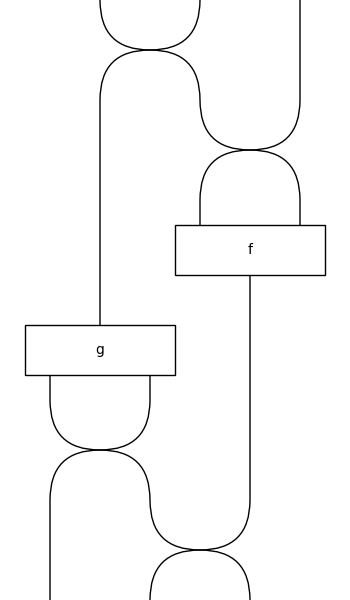# Diagram#

class discopy.symmetric.Diagram(inside, dom, cod, _scan=True)[source]#

A symmetric diagram is a balanced diagram with `Swap` boxes.

Parameters:
• inside (Layer) – The layers inside the diagram.

• dom (monoidal.Ty) – The domain of the diagram, i.e. its input.

• cod (monoidal.Ty) – The codomain of the diagram, i.e. its output.

Note

Symmetric diagrams have a class property structure_preserving, that changes the behaviour of equality and hashing. When set to False, two diagrams equal if they are built from the same layers. When set to True, the underlying hypergraphs are used for hashing and equality checking. The default value of structure_preserving is False. >>> x, y = Ty(“x”), Ty(“y”) >>> id_hash = hash(Id(x @ y)) >>> assert Swap(x, y) >> Swap(y, x) != Id(x @ y) >>> Diagram.structure_preserving = True >>> assert Swap(x, y) >> Swap(y, x) == Id(x @ y) >>> assert id_hash != hash(Id(x @ y)) >>> Diagram.structure_preserving = False

Note

Symmetric diagrams can be defined using the standard syntax for functions.

```>>> x = Ty('x')
>>> f = Box('f', x @ x, x)
>>> g = Box('g', x, x @ x)
```
```>>> @Diagram.from_callable(x @ x @ x, x @ x @ x)
... def diagram(x0, x1, x2):
...     x3 = f(x2, x0)
...     x4, x5 = g(x1)
...     return x5, x3, x4
>>> diagram.draw(draw_type_labels=False,
...              path='docs/_static/symmetric/decorator.png')
```Every variable must be used exactly once or this will raise an error.

```>>> from pytest import raises
>>> from discopy.utils import AxiomError
```
```>>> with raises(AxiomError) as err:
...     Diagram.from_callable(x, x @ x)(lambda x: (x, x))
>>> print(err.value)
symmetric.Diagram does not have copy or discard.
```
```>>> with raises(AxiomError) as err:
...     Diagram.from_callable(x, Ty())(lambda x: ())
>>> print(err.value)
symmetric.Diagram does not have copy or discard.
```

Note

As for `discopy.balanced.Diagram`, our symmetric diagrams are traced by default. However now we have that the axioms for trace hold on the nose.

classmethod swap(left, right)[source]#

The diagram that swaps the `left` and `right` wires.

Parameters:
• left (Ty) – The type at the top left and bottom right.

• right (Ty) – The type at the top right and bottom left.

Return type:

Diagram

Note

This calls `balanced.hexagon()` and `braid_factory`.

classmethod permutation(xs, dom=None)[source]#

The diagram that encodes a given permutation.

Parameters:
• xs (list[int]) – A list of integers representing a permutation.

• dom (Optional[Ty]) – A type of the same length as `permutation`, default is `PRO(len(permutation))`.

Return type:

Diagram

permute(*xs)[source]#

Post-compose with a permutation.

Parameters:

xs (int) – A list of integers representing a permutation.

Return type:

Diagram

Examples

```>>> x, y, z = Ty('x'), Ty('y'), Ty('z')
>>> assert Id(x @ y @ z).permute(2, 0, 1).cod == z @ x @ y
```
to_hypergraph()[source]#

Translate a diagram into a hypergraph.

Return type:

Hypergraph

simplify()[source]#

Simplify by translating back and forth to hypergraph.

depth()[source]#

The depth of a symmetric diagram.

Examples

```>>> x = Ty('x')
>>> f = Box('f', x, x)
>>> assert Id(x).depth() == Id().depth() == 0
>>> assert f.depth() == (f @ f).depth() == 1
>>> assert (f @ f >> Swap(x, x)).depth() == 1
>>> assert (f >> f).depth() == 2 and (f >> f >> f).depth() == 3
```
braid_factory#

alias of `Swap`

factory#

alias of `Diagram`

sum_factory#

alias of `Sum`

trace_factory#

alias of `Trace`